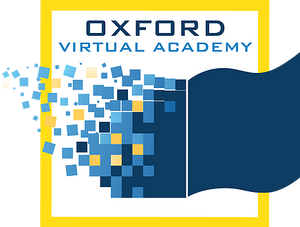# Math 3

This course covers core mathematics concepts, including place value, rounding, estimation, word problems with addition and subtraction, the properties of multiplication, probability, measurement, shapes, patterns, decimals, and comparing fractions. These topics include skills that are necessary to build a foundation in geometry, algebra, trigonometry, and real-world problem solving. Semester 2 covers core mathematics concepts, including place value, rounding, estimation, word problems with addition and subtraction, the properties of multiplication, probability, measurement, shapes, patterns, decimals, and comparing fractions. These topics include skills that are necessary to build a foundation in geometry, algebra, trigonometry, and real-world problem solving.

* Prerequisites: None